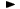ADVFN HomeHelpFinancialsDeeper AnalysisFINANCIAL RATIOS - Leverage RatiosAssets/Equity

# Assets/Equity

Assets to Equity ratio is simply total assets divided by total equity. The formula is the following:

= TOTAL ASSETS / ord cap,reserves + prefs,minorities